#### 1. Extinction Ratio

A simple source of power penalty is related to the energy carried by 0 bits. Some power is emitted by most transmitters even in the off state. In the case of semiconductor lasers, the off-state power P0 depends on the bias current Ib and the threshold current Ith. If Ib < Ith, the power emitted during 0 bits is due to spontaneous emission, and generally P0 << P1 , where P1 is the on-state power. By contrast, P0 can be a significant fraction of P1 if the laser is biased close to but above threshold. The extinction ratio is defined as

rex = P0/P1

The power penalty can be obtained by usingFor a p-i-n receiver I1 = RdP1 and I0 = RdP0 , where Rd is the responsivity (the APD gain can be included by replacing Rd with MRd). By using the definitionfor the receiver sensitivity, the Q factor is given byIn general, σ1 and σ0 depend on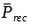because of the dependence of the shot-noise contribution on the received optical signal. However, both of them can be approximated by the thermal noise σT when receiver performance is dominated by thermal noise. By using  σ1 ≈ σ0 ≈ σT in the Q equation above,is given by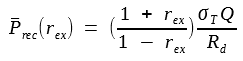This equation shows thatincreases when rex ≠ 0. The power penalty is defined as the ratio. It is commonly expressed in decibel (dB) units by usingThe figure below shows how the power penalty increases with rex.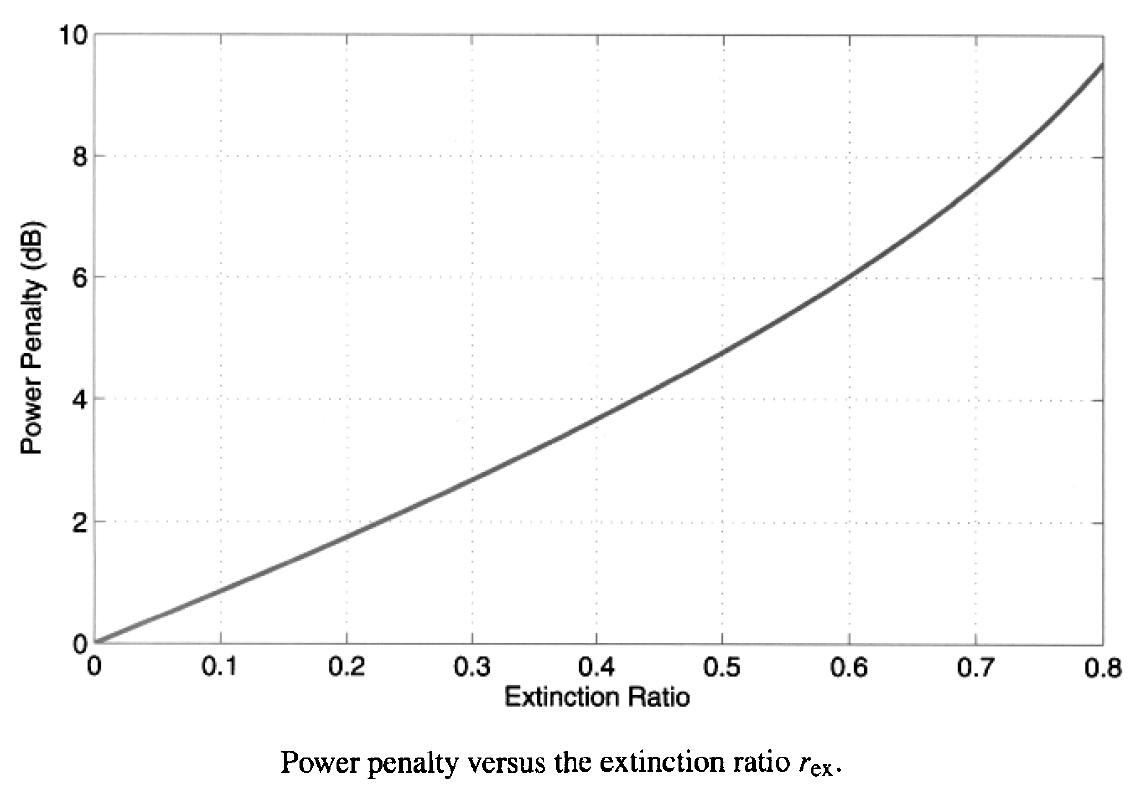A 1-dB penalty occurs for rex = 0.12 and increases to 4.8 dB for rex = 0.5. In practice, for lasers biased below the threshold, rex is typically below 0.05, and the corresponding power penalty (<0.4 dB) is negligible. Nonetheless, it can become significant if the semiconductor laser is biased above threshold. An expression for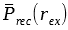can be obtained for APD receivers by including the APD gain and the shot-noise contribution to σ1 and σ0 in the equation above. The optimum APD gain is lower when rex ≠ 0. The sensitivity is also reduced because of the lower optimum gain. Normally, the power penalty for an APD receiver is larger by about a factor of 2 for the same value of rex .

#### 2. Intensity Noise

The noise analysis of the optical receiver in the previous tutorial is based on the assumption that the optical power incident on the receiver does not fluctuate. In practice, light emitted by any transmitter exhibits power fluctuations. Such fluctuations, called intensity noise, were discussed in the context of semiconductor lasers.  The optical receiver converts power fluctuations into current fluctuations which add to those resulting from shot noise and thermal noise. As a result, the receiver SNR is degraded and is lower. An exact analysis is complicated, as it involves the calculation of photocurrent statistics. A simple approach consists of adding a third term to the current variance, so that

σ2 = σs2σT2 + σI2

whereThe parameter rI, defined as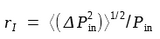, is a measure of the noise level of the incident optical signal. It is related to the relative intensity noise (RIN) of the transmitter as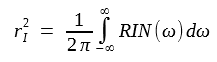rI is simply the inverse of the SNR of light emitted by the transmitter. Typically, the transmitter SNR is better than 20 dB, and rI < 0.01.

As a result of the dependence of σ0 and σ1 on the parameter rI, the parameter Q is reduced in the presence of intensity noise. Since Q should be maintained to the same value to maintain the BER, it is necessary to increase the received power. This is the origin of the power penalty induced by intensity noise. To simplify the following analysis, the extinction ratio is assumed to be zero, so that I0 = 0 and σ0 = σT. By usingand σ2 equation above for σ1, Q is given by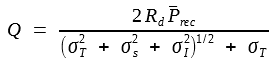where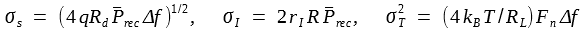This Q equation is easily solved to obtain the following expression for the receiver sensitivity: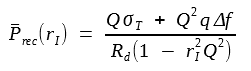The power penalty, defined as the increase in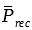when rI ≠ 0, is given byThe following figure shows the power penalty as a function of rI for maintaining Q = 6 and 7 corresponding to a BER of 10-9 and 10-12, respectively.The penalty is negligible for rI < 0.01 as δI is below 0.02 dB. Since this is the case for most practical optical transmitters, the effect of transmitter noise is negligible in practice. The power penalty exceeds 2 dB for rI = 0.1 and becomes infinite when rI = 1/Q. An infinite power penalty implies that the receiver cannot operate at the specific BER even if the incident optical power is increased indefinitely. In the case of Bit-error rate versus the Q parameter, an infinite power penalty corresponds to a saturation of the BER curve above the 10-9 level for Q = 6, a feature referred to as the BER floor. In this respect, the effect of intensity noise is qualitatively different than the extinction ratio, for which the power penalty remains finite for all values of rex such that rex < 1.

The preceding analysis assumes that the intensity noise at the receiver is the same as at the transmitter. This is not typically the case when the optical signal propagates through a fiber link. The intensity noise added by in-line optical amplifiers often becomes a limiting factor for most long-haul lightwave systems. When a multimode semiconductor laser is used, fiber dispersion can lead to degradation of the receiver sensitivity through the mode-partition noise. Another phenomenon that can enhance intensity noise is optical feedback from parasitic reflections occurring all along the fiber link. Such transmission-induced power-penalty mechanisms are considered in another tutorial.

#### 3. Timing Jitter

The calculation of receiver sensitivity is based on the assumption that the signal is sampled at the peak of the voltage pulse. In practice, the decision instant is determined by the clock-recovery circuit. Because of the noisy nature of the input to the clock-recovery circuit, the sampling time fluctuates from bit to bit. Such fluctuations are called timing jitter. The SNR is degraded because fluctuations in the sampling time lead to additional fluctuations in the signal. This can be understood by noting that if the bit is not sampled at the bit center, the sampled values is reduced by an amount that depends on the timing jitter Δt. Since Δt is a random variable, the reduction in the sampled value is also random. The SNR is reduced as a result of such additional fluctuations, and the receiver performance is degraded. The SNR can be maintained by increasing the received optical power. This increase is the power penalty induced by timing jitter.

To simplify the following analysis, let us consider a p-i-n receiver dominated by thermal noise σT and assume a zero extinction ratio. By using I0 = 0, the Q factor is given bywhere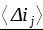is the average value and σj is the RMS value of the current fluctuation Δtj induced by timing jitter Δt. If hout(t) governs the shape of the current pulse,

Δtj = I1[hout(0) - hout(Δt)]

where the ideal sampling instant is taken to be t = 0.

Clearly, σj depends on the shape of the signal pulse at the decision current. A simple choice corresponds to hout(t) = cos2(πBt/2), where B is the bit rate. Here the following is used as many optical receivers are designed to provide that pulse shape.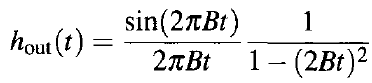Since Δt is likely to be much smaller than the bit period TB  = 1/B, it can be approximated asby assuming that BΔt << 1. This approximation provides a reasonable estimate of the power penalty as long as the penalty is not too large. This is expected to be the case in practice. To calculate σj, the probability density function of the timing jitter Δt is assumed to be Gaussian, so that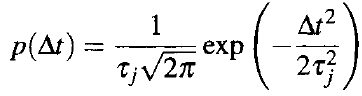where τj is the RMS value (standard deviation) of Δt. The probability density of Δtj can be obtained by using the two equations above and noting that Δtj is proportional to (Δt)2. The result is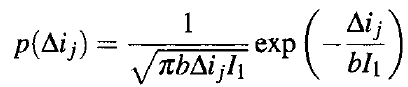where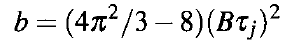The equation above is used to calculate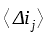and. The integration over Δij is easily done to obtain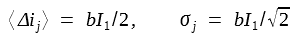Noting that, where Rd is the responsivity, the receiver sensitivity is given by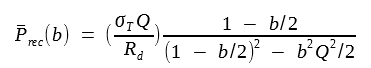The power penalty, defined as the increase in, is given byThe following figure shows how the power penalty varies with the parameter Bτj, which has the physical significance of the fraction of the bit period over which the decision time fluctuates (one standard deviation).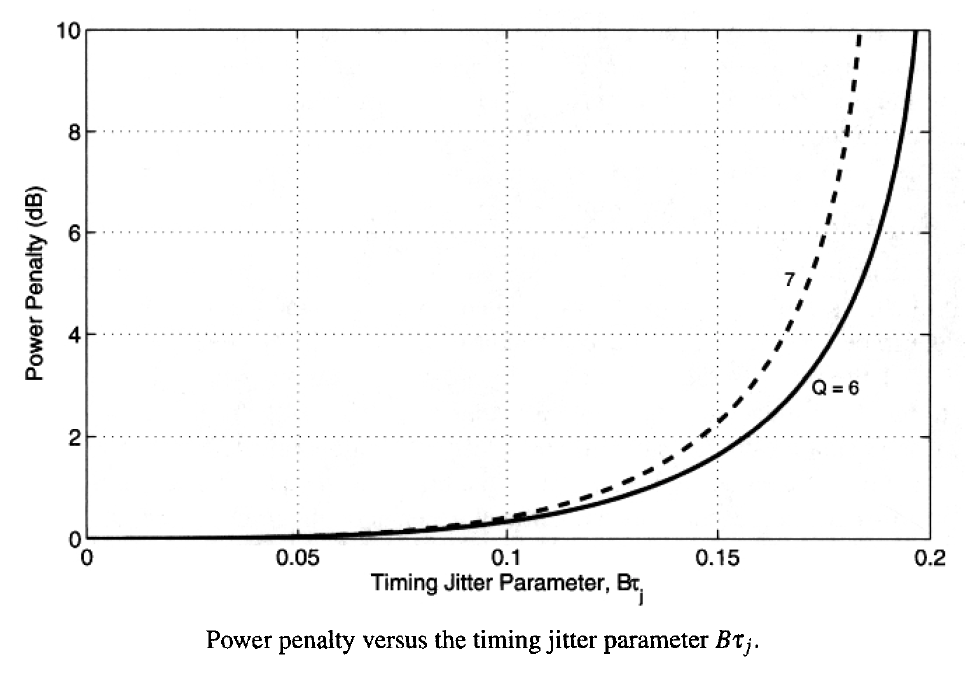The power penalty is negligible for Bτj < 0.1 but increases rapidly beyond Bτj = 0.1. A 2-dB penalty occurs for Bτj = 0.16. Similar to the case of intensity noise, the jitter-induced  penalty becomes infinite beyond Bτj = 0.2. The exact value of Bτj at which the penalty becomes infinite depends on the model used to calculate the jitter-induced power penalty. The δj equation above is obtained by using a specific pulse shape and a specific jitter distribution. It also assumes Gaussian statistics for the receiver current. Jitter-induced current fluctuations are not Gaussian in nature. A ore accurate calculation shows that the δj equation above underestimates the power penalty. The qualitative behavior, however, remains the same. In general, the RMS value of the timing jitter should be below 10% of the bit period for a negligible power penalty. A similar conclusion holds ofr APD receivers, for which the penalty is generally larger